# Least Common Multiple

The least common multiple of two integers a and b is the smallest positive integer that is divisible by both a and b. By “least,” we mean “smallest” or “lowest” common multiple. For this explanation, we will refer to the least common multiple of a and b as LCM(a, b), but the notation “LCM” is not something you need to know for GMAT questions.

On the GMAT, some questions might ask for the LCM directly. In other questions, you will need to add fractions and can find the LCM handy as a common denominator for those fractions. On yet other questions, you will not need to compute the LCM but the kind of thinking that we are discussing here will be central to the question.

A multiple of a number is the product of that number and an integer. For example, 10 is a multiple of 5 because 5 times 2 is 10, so 10 is divisible by 5 and 2. Because 10 is the smallest positive integer that is divisible by both 5 and 2, it is the least common multiple of 5 and 2.

Example: What is the LCM of 4 and 6?

The multiples of 4 are: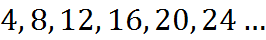and the multiples of 6 are: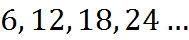Common multiples of 4 and 6 are simply the numbers that are in both lists: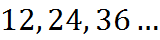The least common multiple is the smallest member of this list of common multiples. So, the LCM of 4 and 6 is 12.

When adding, subtracting, or comparing fractions, we require a common denominator. This is discussed in the section on Fractions in this book. One way to get a common denominator is to find the LCM of the denominators. For instance,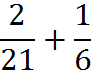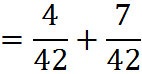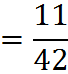In this example, we need a common denominator to add the fractions. The LCM of 21 and 6 is 42, which is therefore an option for a common denominator.

## Finding the LCM by Prime Factorization

We can find the LCM of a set of numbers using prime factorizations, which we have discussed above. For example, the prime factorization of 90 is: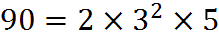We can think of the composite number 90 as made up of one “atom” of the prime number 2, two “atoms” of the prime number 3 and one “atom” of the prime number 5.

To use prime factorizations to find the LCM of a set of numbers, we find the prime factorizations of all the numbers involved and construct the LCM out of the minimum group of prime factors that will have all the necessary “atoms” to create each number in the set.

Example: Let’s find the value of LCM(8,9,21). First, find the prime factorization of each of the numbers: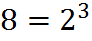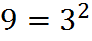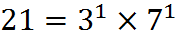The LCM will be the product of each prime number taken to the highest power that it appears in any of the numbers in the set. For example, for LCM(8,9,21) to include 8 as a factor, it must include at least three 2’s as factors. Thus,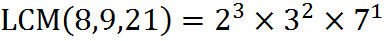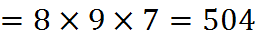This method can be illustrated using a Venn diagram. In another example, let’s find the LCM of 48 and 180. First, again, we find the prime factorizations of 48 and 180: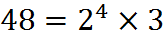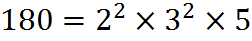Then, we can put the prime factors into a Venn diagram with one circle for each of the two numbers, and all factors they share in common in the intersection. We get the following diagram: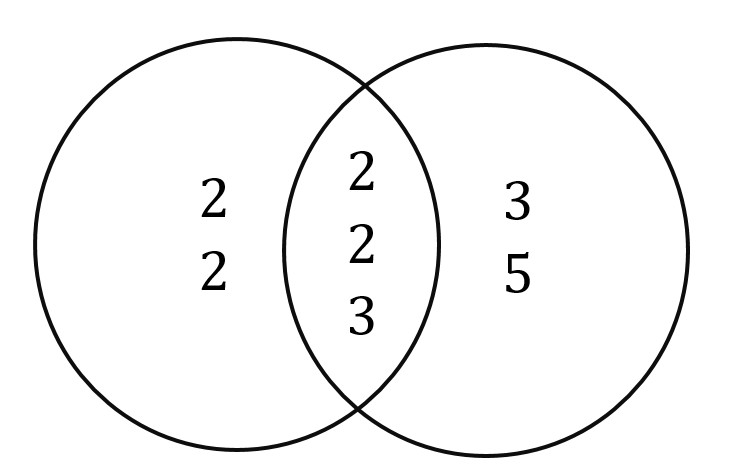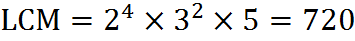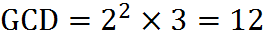The LCM is the product of all of the numbers in the diagram. This diagram also shows us the greatest common divisor (GCD) between the two numbers. The GCD, which is the biggest number that will go into 48 and 180, is the product of the intersection of the prime factors. Thus the GCD of 48 and 180 is 12. The LCM is the union of the sets; the GCD is the intersection. We will discuss unions, intersections, and Venn diagrams in more detail later in this book.

## Practice Questions

Least Common Multiple:
http://www.gmatfree.com/least-common-multiple

Fractions of Inventory:
http://www.gmatfree.com/fractions-of-inventory

Welcome! You are encouraged to register with the site and login (for free). When you register, you support the site and your question history is saved.